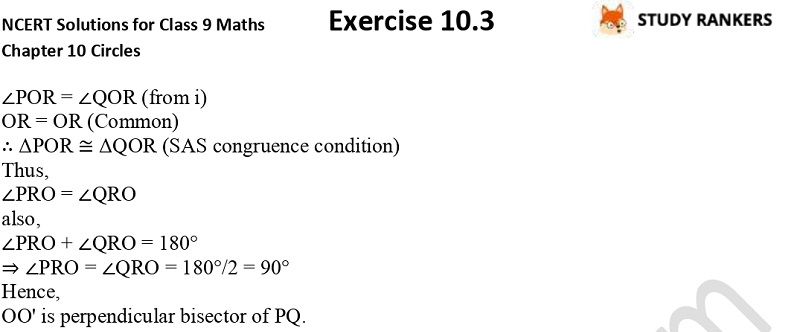>

NCERT Solutions for Class 9 Maths Chapter 10 Circles Exercise 10.3

You can find NCERT Solutions for Class 9 Maths Chapter 10 Circles Exercise 10. 3 here which are prepared by subject matter experts of Studyrankers. Class 9 Maths NCERT Solutions provided here will guide in developing your problem solving skills and knowing the basic concepts given in this exercise. You will get to know about important formulas that will help you in solving the questions in higher classes also. NCERT questions and answers can be helpful in you're running late in completing homework.

Exercise 10.3 has total three questions in which you have to prove that their centres lie on the perpendicular bisector of the common chord etc.X